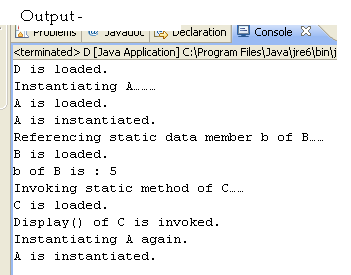• www.uptuexam.com
• GBTU Results 2014
• MTU Results 2014
• UPTU Circulars
• COP Result 2012,13,14
• UPTU Previous Papers
• GATE study material
• Robotics Projects
Thank you for visiting www.UPTUexam.com " Free download Exam materials, previous year question papers, free ebooks & Providing Important Questions To Do their Exams Well and Totally a FREE SERVICE for all engineering streams (B.Tech)."

### At the time of class loading following sequence of steps is performed by JRE

##### At the time of class loading following sequence of steps is performed by JRE

(1). Static Data Members (if defined) are created in the Class Area.
(2). Static Initializer Block (if defined) is executed.
(3). Reference of the class which resulted in class loading is resolved i.e. operation represented by           the reference is performed.

1. class A
2. {
3. static
4. {
6. }
7. public A()
8. {
9. System.out.println(“A is instantiated.”);
10. }
11. }

12. class B
13. {
14. static int b;
15. static
16. {
17. b=5;
19. }
20. }

21. class C
22. {
23. static
24. {
26. }
27. public static void display( )
28. {
29. System.out.println(“Display( ) of C is invoked.”);
30. }
31. }
32. class D
33. {
34. static
35. {
37. }
38. public static void main(String args[])
39. {
40. System.out.println(“Instantiating A………”);
41. A x = new A();
42. System.out.println(“Referencing static data member b of B……”);
43. System.out.println(“b of B is : “ + B.b);
44. System.out.println(“Invoking static method of C……”);
45. C.display();
46. System.out.println(“Instantiating A again.”);
47. A y = new A();
48. }
49. }
50.class E
{
static int a = 5;

public static void main(String args[])
{
System.out.println(“a = “ + a);
}

 After compilation
}

class E
{
static int a;

static
{
a = 5;
}
----
----
}
-------------------------------------------------------------------------------------------------------

class E
{
int a = 5, b=6;

public static void main(String args[])
{
E x = new E();
System.out.println(“a = “ + x.a);
System.out.println(“b = “ + x.b);

}
 After compilation
}

class E
{

int a, b;

E( )
{
a = 5;
b = 6;
}
----
----
}
-------------------------------------------------------------------------------------------------------
class E
{
int a=5, b=6;

public E ()
{
System.out.println(“Default.”);
}

public E(int x)
{
a=x;
System.out.println(“One parameterized.”);
}

public E(int x, int y)
{
b=y;
System.out.println(“Two parameterized.”);
}

public void display()
{
System.out.println(“a = “ + a);
System.out.println(“b = “ + b);
}

public static void main(String args[])
{
E x = new E();
E y = new E(10);
E z = new E(40, 50);
x.display();
y.display();
z.display();
}

}After compilation,

the compiler will do the following for the same program

1. class E
{
int a, b;

public E()
{
a=5, b=6;
System.out.println(“Default.”);
}

public E(int x)
{
a=5, b=6;
a=x;
System.out.println(“One parameterized.”);
}

public E(int x, int y)
{
a=5, b=6;
b=y;
System.out.println(“Two parameterized.”);
}

public void display()
{
System.out.println(“a = “ + a);
System.out.println(“b = “ + b);
}

public static void main(String args[])
{
E x = new E();
E y = new E(10);
E z = new E(40, 50);
x.display();
y.display();
z.display();
}
}